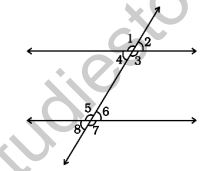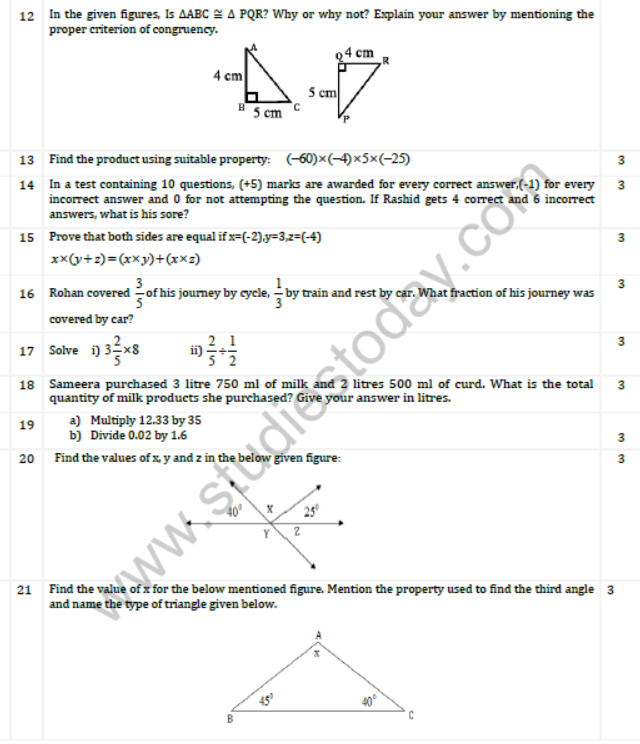# CBSE Class 7 Mathematics Sample Paper Set B

Read and download PDF of CBSE Class 7 Mathematics Sample Paper Set B designed as per the latest curriculum and examination pattern for Class 7 issued by CBSE, NCERT and KVS. The latest Class 7 Mathematics Sample Papers have been provided with solutions so that the students can solve these practice papers and then compare their answers. This will help them to identify mistakes and improvement areas in Mathematics Standard 7 which they need to study more to get better marks in Grade 7 exams. After solving these guess papers also refer to solved Class 7 Mathematics Question Papers available on our website to build strong understanding of the subject

## Sample Paper for Class 7 Mathematics Pdf

Students can refer to the below Class 7 Mathematics Sample Paper designed to help students understand the pattern of questions that will be asked in Grade 7 exams. Please download CBSE Class 7 Mathematics Sample Paper Set B

### Mathematics Class 7 Sample Paper

CBSE Class 7 Mathematics Sample Paper Set B. It’s always recommended to practice as many CBSE sample papers as possible before the examinations. The latest sample papers have been designed as per the latest blue prints, syllabus and examination trends. Sample papers should be practiced in examination condition at home or school and also show it to your teachers for checking or compare with the answers provided. Students can download the sample papers in pdf format free and score better marks in examinations. Refer to other links too for latest sample papers.

1 Subtract (-2) from 15

2 Convert 120.33 g into kg.

3 Write the algebraic equation for the following statement: Subtract the product of 2 and x from 7.

4 Indicate two different pairs of corresponding angles in the given figure?5 If two angles of a triangle are 60° each, then the triangle is

6 How many altitudes does a triangle have?

7 Evaluate the following, using distributive property:

(-876)x9+(-876)

8.Write four equivalent fractions of 2/5

9 Form the equation and then solve : The product of 5 and x is 20

10 If the supplement of an angle is 1690  , Find the angle.

11 If the measure of two angles of a triangle are 35and 650 respectively. Find the remaining angle.

Also mention the name of the property used.